# CBSE Class 11 Mathematics Conic Sections Notes

Download CBSE Class 11 Mathematics Conic Sections Notes in PDF format. All Revision notes for Class 11 Mathematics have been designed as per the latest syllabus and updated chapters given in your textbook for Mathematics in Class 11. Our teachers have designed these concept notes for the benefit of Class 11 students. You should use these chapter wise notes for revision on daily basis. These study notes can also be used for learning each chapter and its important and difficult topics or revision just before your exams to help you get better scores in upcoming examinations, You can also use Printable notes for Class 11 Mathematics for faster revision of difficult topics and get higher rank. After reading these notes also refer to MCQ questions for Class 11 Mathematics given on studiestoday

## Revision Notes for Class 11 Mathematics Chapter 11 Conic Sections

Class 11 Mathematics students should refer to the following concepts and notes for Chapter 11 Conic Sections in Class 11. These exam notes for Class 11 Mathematics will be very useful for upcoming class tests and examinations and help you to score good marks

### Chapter 11 Conic Sections Notes Class 11 Mathematics

Key Concepts

1. Let l  be a fixed vertical line and m be another line intersecting it at a fixed point V and inclined to it at and angle a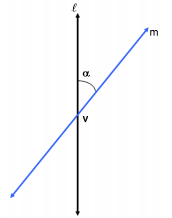On rotating the line m around the line l in such a way that the angle a remains constant a surface is generated is a double-napped right circular hollow cone.2. The point V is called the vertex; the line l is the axis of the cone. The rotating line m is called a generator of the cone. The vertex separates the cone into two parts called nappes.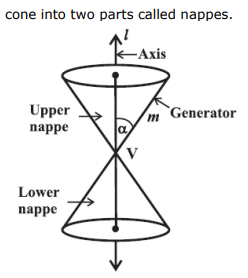3. The sections obtained by cutting a double napped cone with a plane are called conic sections. Conic sections are of two types (i) degenerate conics (ii) non degenerate conics.

4. If the cone is cut at its vertex by the plane then degenerate conics are obtained.

5. If the cone is cut at the nappes by the plane then non degenerate conics are obtained.

6. Degenerate conics are point, line and double lines.

7. Circle, parabola, ellipse and hyperbola are degenerate conics.

8. When the plane cuts the nappes (other than the vertex) of the cone,degenerate conics are obtained.

(a) When β = 90o , the section is a circle.The plane cuts the cone horizontally.

(b) When α < β < 90o, the section is an ellipse.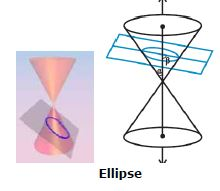The plane cuts one part of the cone in an inclined manner

(c) When β = α; the section is a parabola.The plane cuts the cone in such a way that it is parallel to a generator

(d) When 0 ≤  β < α; the plane cuts through both the nappes the curve of intersection is a hyperbola.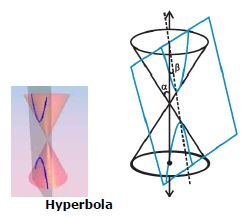The plane cuts both parts of the cone.

9. When the plane cuts at the vertex of the cone, we have the following different cases:

(a) When α <β  900, then the section is a point.(b) When β = α, the plane contains a generator of the cone and the section is a straight line.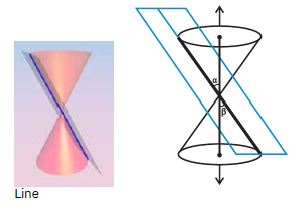It is the degenerated case of parabola.

 CBSE Class 11 Mathematics Set Theory Notes
 CBSE Class 11 Mathematics Relations And Functions Notes
 CBSE Class 11 Mathematics Complex Numbers Quadratic Equations Notes
 CBSE Class 11 Mathematics Linear Inequalities Notes
 CBSE Class 11 Mathematics Permutation And Combination Formulas And Questions CBSE Class 11 Mathematics Permutation And Combination Notes
 CBSE Class 11 Mathematics Sequence And Series Formulas And Questions CBSE Class 11 Mathematics Sequence And Series Notes
 CBSE Class 11 Mathematics Straight Lines Formulas And Questions
 CBSE Class 11 Mathematics Coordinate Geometry Formulas And Questions CBSE Class 11 Mathematics Three Dimensional Geometry Notes
 CBSE Class 11 Mathematics Limits And Derivatives Notes
 CBSE Class 11 Mathematics Mathematical Reasoning Notes
 CBSE Class 11 Mathematics Statistics Notes
 CBSE Class 11 Mathematics Probability Notes

## More Study Material

### CBSE Class 11 Mathematics Chapter 11 Conic Sections Notes

We hope you liked the above notes for topic Chapter 11 Conic Sections which has been designed as per the latest syllabus for Class 11 Mathematics released by CBSE. Students of Class 11 should download and practice the above notes for Class 11 Mathematics regularly. All revision notes have been designed for Mathematics by referring to the most important topics which the students should learn to get better marks in examinations. Studiestoday is the best website for Class 11 students to download all latest study material.

### Notes for Mathematics CBSE Class 11 Chapter 11 Conic Sections

Our team of expert teachers have referred to the NCERT book for Class 11 Mathematics to design the Mathematics Class 11 notes. If you read the concepts and revision notes for one chapter daily, students will get higher marks in Class 11 exams this year. Daily revision of Mathematics course notes and related study material will help you to have a better understanding of all concepts and also clear all your doubts. You can download all Revision notes for Class 11 Mathematics also from www.studiestoday.com absolutely free of cost in Pdf format. After reading the notes which have been developed as per the latest books also refer to the NCERT solutions for Class 11 Mathematics provided by our teachers

#### Chapter 11 Conic Sections Notes for Mathematics CBSE Class 11

All revision class notes given above for Class 11 Mathematics have been developed as per the latest curriculum and books issued for the current academic year. The students of Class 11 can rest assured that the best teachers have designed the notes of Mathematics so that you are able to revise the entire syllabus if you download and read them carefully. We have also provided a lot of MCQ questions for Class 11 Mathematics in the notes so that you can learn the concepts and also solve questions relating to the topics. All study material for Class 11 Mathematics students have been given on studiestoday.

#### Chapter 11 Conic Sections CBSE Class 11 Mathematics Notes

Regular notes reading helps to build a more comprehensive understanding of Chapter 11 Conic Sections concepts. notes play a crucial role in understanding Chapter 11 Conic Sections in CBSE Class 11. Students can download all the notes, worksheets, assignments, and practice papers of the same chapter in Class 11 Mathematics in Pdf format. You can print them or read them online on your computer or mobile.

#### Notes for CBSE Mathematics Class 11 Chapter 11 Conic Sections

CBSE Class 11 Mathematics latest books have been used for writing the above notes. If you have exams then you should revise all concepts relating to Chapter 11 Conic Sections by taking out a print and keeping them with you. We have also provided a lot of Worksheets for Class 11 Mathematics which you can use to further make yourself stronger in Mathematics

Where can I download latest CBSE Class 11 Mathematics Chapter 11 Conic Sections notes

You can download notes for Class 11 Mathematics Chapter 11 Conic Sections for latest academic session from StudiesToday.com

Can I download the Notes for Chapter 11 Conic Sections Class 11 Mathematics in Pdf format

Yes, you can click on the link above and download notes PDFs for Class 11 Mathematics Chapter 11 Conic Sections which you can use for daily revision

Are the revision notes available for Chapter 11 Conic Sections Class 11 Mathematics for the latest CBSE academic session

Yes, the notes issued for Class 11 Mathematics Chapter 11 Conic Sections have been made available here for latest CBSE session

How can I download the Chapter 11 Conic Sections Class 11 Mathematics Notes pdf

You can easily access the link above and download the Class 11 Notes for Mathematics Chapter 11 Conic Sections for each topic in Pdf

Is there any charge for the Class 11 Mathematics Chapter 11 Conic Sections notes

There is no charge for the notes for CBSE Class 11 Mathematics Chapter 11 Conic Sections, you can download everything free of charge

Which is the best online platform to find notes for Chapter 11 Conic Sections Class 11 Mathematics

www.studiestoday.com is the best website from which you can download latest notes for Chapter 11 Conic Sections Mathematics Class 11

Where can I find topic-wise notes for Class 11 Mathematics Chapter 11 Conic Sections

Come to StudiesToday.com to get best quality topic wise notes for Class 11 Mathematics Chapter 11 Conic Sections

Can I get latest Chapter 11 Conic Sections Class 11 Mathematics revision notes as per CBSE syllabus

We have provided all notes for each topic of Class 11 Mathematics Chapter 11 Conic Sections as per latest CBSE syllabus